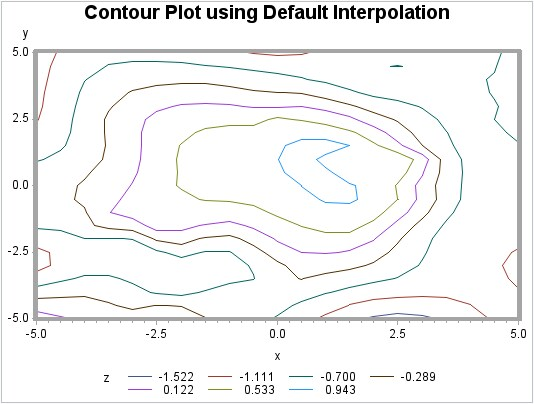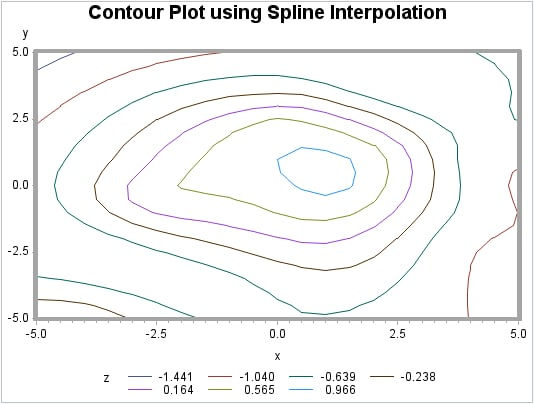# Example 4: Spline Interpolation

 Features: GRID statement options AXIS1=, AXIS2=, and SPLINE Data set: NUMS Sample library member: GTGSPLIN
This example demonstrates the default interpolation method when used by the GCONTOUR procedure to generate a contour plot from the resulting output data set.
Contour Plot Using Default Interpolation (gtgsplin)The second plot, demonstrates the spline interpolation method when used by the GCONTOUR procedure to generate a contour plot from the resulting output data set.
Contour Plot Using Spline Interpolation (gtgsplin)## Program

`goptions reset=all border;`
```title "Contour Plot using Default
Interpolation";```
`axis1 width=3;`
```proc g3grid data=nums out=numdef;
grid y*x=z /
axis1=-5 to 5 by .5
axis2=-5 to 5 by .5;
run;```
```proc gcontour data=numdef;
plot y*x=z /
haxis=axis1
vaxis=axis1;
run;
quit;```
```title "Contour Plot using Spline
Interpolation";```
```proc g3grid data=nums out=numspl;
grid y*x=z / spline
axis1=-5 to 5 by .5
axis2=-5 to 5 by .5;
run;```
```proc gcontour
data=numspl;
plot y*x=z /
haxis=axis1
vaxis=axis1;
run;
quit;```

## Program Description

Set the graphics environment.
`goptions reset=all border;`
Define the title for the plot.
```title "Contour Plot using Default
Interpolation";```
Define the axis characteristics.
`axis1 width=3;`
Process data with PROC G3GRID.
```proc g3grid data=nums out=numdef;
grid y*x=z /
axis1=-5 to 5 by .5
axis2=-5 to 5 by .5;
run;```
Generate the contour after default interpolation.
```proc gcontour data=numdef;
plot y*x=z /
haxis=axis1
vaxis=axis1;
run;
quit;```
Define the title for the plot.
```title "Contour Plot using Spline
Interpolation";```
Process data with PROC G3GRID. The SPLINE option specifies the bivariate spline method for the interpolation.
```proc g3grid data=nums out=numspl;
grid y*x=z / spline
axis1=-5 to 5 by .5
axis2=-5 to 5 by .5;
run;```
Generate the contour plot using the spline interpolation.
```proc gcontour
data=numspl;
plot y*x=z /
haxis=axis1
vaxis=axis1;
run;
quit;```